# 06-1350/Class Notes for Thursday October 12

## A Question

Determine the image of the differential operator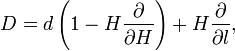$D=d\left(1-H\frac{\partial}{\partial H}\right)+H\frac{\partial}{\partial l},$

acting on polynomials in the variables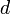$d$,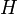$H$ and$l$. More precisely - determine the quotient of the space of polynomials in$d$,$H$ and$l$ by the image of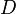$D$. More generally, it is a standard subject in algebra to deal with the images and cokernels of algebraic operators; the results are ideals and the quotients are often-studied algebras. But where's the theory for the images and "cokernels" of differential operators.

### Why do I care?

The answer to this question is directly related to the determination of the "envelope" of the Alexander polynomial for two component links.

## Some Computations

The following table (taken from and ) shows the number of type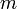$m$ invariants of knots and framed knots modulo type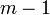$m-1$ invariants (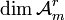$\dim{\mathcal A}_m^r$ and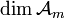$\dim{\mathcal A}_m$) and the number of multiplicative generators of the algebra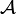${\mathcal A}$ in degree$m$ (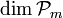$\dim{\mathcal P}_m$) for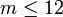$m\leq 12$. Some further tabulated results are in .

 m 0 1 2 3 4 5 6 7 8 9 10 11 12$\dim{\mathcal A}_m^r$ 1 0 1 1 3 4 9 14 27 44 80 132 232$\dim{\mathcal A}_m$ 1 1 2 3 6 10 19 33 60 104 184 316 548$\dim{\mathcal P}_m$ 0 1 1 1 2 3 5 8 12 18 27 39 55

## Some Further Computations

Some further computations for links and tangles were made by Siddarth Sankaran and Zavosh Amir-Khosravi. See VasCalc Results - ChordMod4T.

## Some Bounds

Little is known about these dimensions for large$m$. There is an explicit conjecture in but no progress has been made in the direction of proving or disproving it. The best asymptotic bounds available are:

Theorem. For large$m$,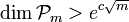$\dim{\mathcal P}_m>e^{c\sqrt m}$ (for any fixed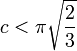$c<\pi\sqrt{\frac23}$, see , ) and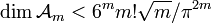$\dim{\mathcal A}_m<6^mm!\sqrt{m}/\pi^{2m}$ (, ).

## Some References

[Bar-Natan_95] ^  D. Bar-Natan, On the Vassiliev knot invariants, Topology 34 (1995) 423-472.

[Bar-Natan_96] ^  D. Bar-Natan, Some computations related to Vassiliev invariants, electronic publication, http://www.math.toronto.edu/~drorbn/LOP.html#Computations.

[Broadhurst_97] ^  D. J. Broadhurst, Conjectured enumeration of Vassiliev invariants, preprint, September 1997, arXiv:q-alg/9709031.

[Dasbach_00] ^  O. T. Dasbach, On the combinatorial structure of primitive Vassiliev invariants III - a lower bound, Comm. in Cont. Math. 2-4 (2000) 579-590, arXiv:math.GT/9806086.

[Kneissler_97] ^  J. A. Kneissler, The number of primitive Vassiliev invariants up to degree twelve, preprint, June 1997, arXiv:q-alg/9706022.

[Kontsevich] ^  M. Kontsevich, unpublished.

[Stoimenow_98] ^  A. Stoimenow, Enumeration of chord diagrams and an upper bound for Vassiliev invariants, Jour. of Knot Theory and its Ramifications 7(1) (1998) 94-114.

[Zagier_01] ^  D. Zagier, Vassiliev invariants and a strange identity related to the Dedekind eta-function, Topology 40(5) (2001) 945-960.